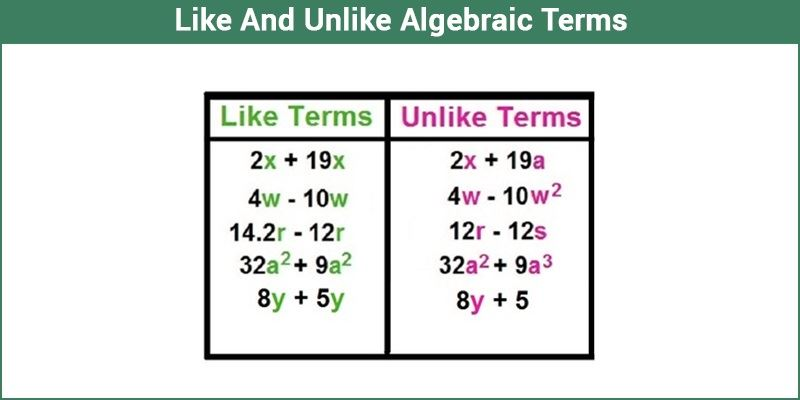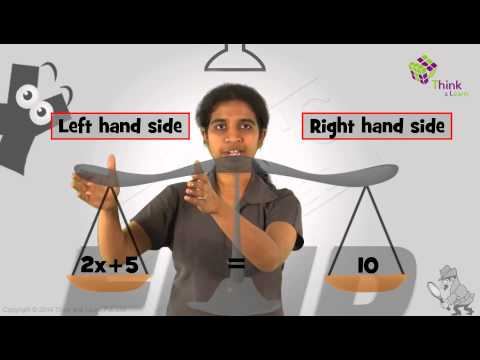Checkout JEE MAINS 2022 Question Paper Analysis : Checkout JEE MAINS 2022 Question Paper Analysis :

# Like and Unlike Algebraic Terms

Before getting into like terms and unlike terms, one should be able to distinguish what an algebraic term is? You can understand this by using an example. 9x + 4y² = 5 is an algebraic equation. It consists of 3 terms, i.e. 9x, 4y² and 5. The first two terms consist of variables and 5 is a constant. 9x + 4y² is an algebraic expression. It has two terms 9x and 4y². In this article, you are going to learn the definition of like and unlike algebraic terms in Maths with more examples.## What are Like Terms?

In Algebra, the like terms are defined as the terms that contain the same variable which is raised to the same power. In algebraic like terms, only the numerical coefficients can vary. We can combine the like terms to simplify the algebraic expressions so that the result of the expression can be obtained very easily.

For example, 4x + 10x is an algebraic expression with like terms. In order to simplify this algebraic expression, we can add the like terms. Thus, the simplification of the given expression is 14x. In the same way, you can perform all the arithmetic operations on the like terms.

## What are Unlike Terms?

Algebraic terms, which does not have the same literal coefficients, and cannot be raised to the same power are called, unlike terms.

For example, 4x + 9y is an algebraic expression with unlike terms. Because it has two different variables x and y, and not raised to the same power.

### Like and Unlike  Algebraic Terms Examples

Algebraic terms are those individual elements in an equation or an expression separated by ‘+’ or ‘-’ signs.

Consider the expression: 9x + 6y

We cannot simplify this any further as ‘x’ and ‘y’ are unknown. Check out another example.

4x² + 3x + 4y + 8x + 10x²

If rearranged, this expression can be re-written as:

10x² + 4x²+ 8x + 3x + 4y

= 14x² + 11x + 4y

Thus, it is seen that algebraic terms with the same variables are added to each other. The addition of certain terms was possible only because the variables in both these cases are the same even if the numerical coefficients are different which can be added as normal numbers and the variable factor remains as it is. Now, these terms which have the same variables are called like terms.

Thus, terms having identical variables raised to the same exponent are like terms.

So, what is 14x², 11x and 4y called? These are called unlike terms since the variables or exponent raised to these variables are “unlike” or not same.

Further operations on unlike terms cannot be directly performed. Based on this, it is clear that an algebraic expression consists of terms which can be categorized into like terms and unlike terms.

Let’s consider another example 2xy + 4x² + 5xy +5y² +16x²

If you notice properly, the terms 2xy and 5xy, as well as 4x²and 16 x² have common factors. Only the numerical coefficients are different. Apart from that, all the variable factors are the same, so these terms can be added.

2xy + 5xy = 7xy

4x² +16x² = 20 x²

Because these terms have the variable factors in common and an arithmetic operation can be performed on them, they are called like terms. After adding the like terms, consider the expression again.

2xy + 4x² + 5xy + 5y² +16x² = 7xy + 20x² + 5y²

If you observe the expression now, none of the terms has any common factors and no arithmetic operation can be performed on them further. These terms are called, unlike terms.